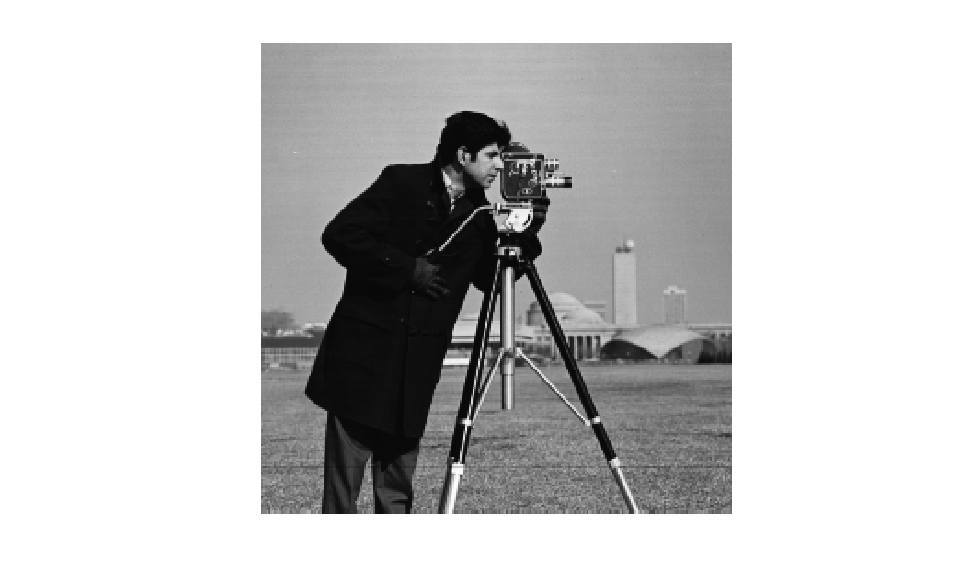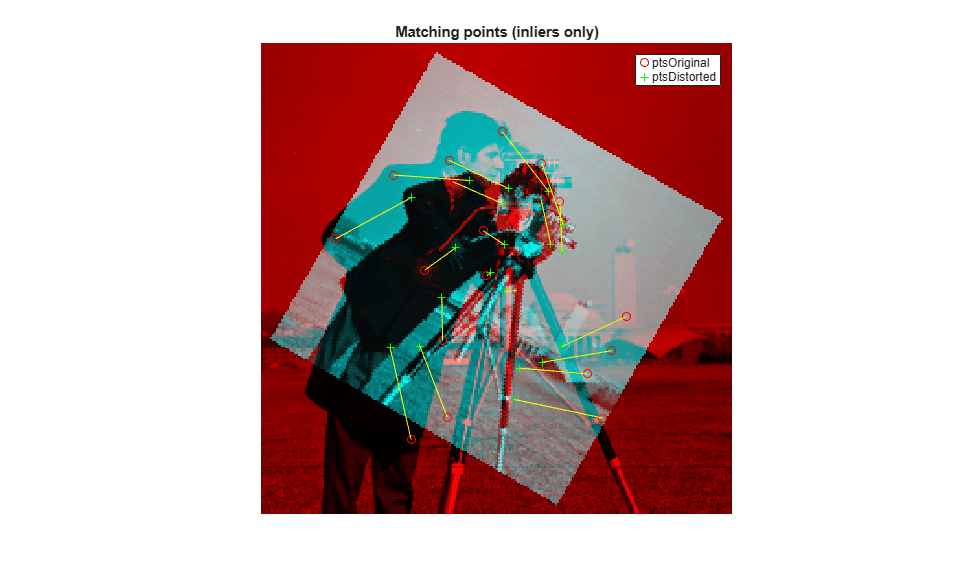Documentation

This is machine translation

Mouseover text to see original. Click the button below to return to the English version of the page.

Find Image Rotation and Scale Using Automated Feature Matching

This example shows how to automatically determine the geometric transformation between a pair of images. When one image is distorted relative to another by rotation and scale, use `detectSURFFeatures` and `estimateGeometricTransform` to find the rotation angle and scale factor. You can then transform the distorted image to recover the original image.

Bring an image into the workspace.

```original = imread('cameraman.tif'); imshow(original); text(size(original,2),size(original,1)+15, ... 'Image courtesy of Massachusetts Institute of Technology', ... 'FontSize',7,'HorizontalAlignment','right'); ```Step 2: Resize and Rotate the Image

```scale = 0.7; J = imresize(original, scale); % Try varying the scale factor. theta = 30; distorted = imrotate(J,theta); % Try varying the angle, theta. figure, imshow(distorted) ```You can experiment by varying the scale and rotation of the input image. However, note that there is a limit to the amount you can vary the scale before the feature detector fails to find enough features.

Step 3: Find Matching Features Between Images

Detect features in both images.

```ptsOriginal = detectSURFFeatures(original); ptsDistorted = detectSURFFeatures(distorted); ```

Extract feature descriptors.

```[featuresOriginal, validPtsOriginal] = extractFeatures(original, ptsOriginal); [featuresDistorted, validPtsDistorted] = extractFeatures(distorted, ptsDistorted); ```

Match features by using their descriptors.

```indexPairs = matchFeatures(featuresOriginal, featuresDistorted); ```

Retrieve locations of corresponding points for each image.

```matchedOriginal = validPtsOriginal(indexPairs(:,1)); matchedDistorted = validPtsDistorted(indexPairs(:,2)); ```

Show putative point matches.

```figure; showMatchedFeatures(original,distorted,matchedOriginal,matchedDistorted); title('Putatively matched points (including outliers)'); ```Step 4: Estimate Transformation

Find a transformation corresponding to the matching point pairs using the statistically robust M-estimator SAmple Consensus (MSAC) algorithm, which is a variant of the RANSAC algorithm. It removes outliers while computing the transformation matrix. You may see varying results of the transformation computation because of the random sampling employed by the MSAC algorithm.

```[tform, inlierDistorted, inlierOriginal] = estimateGeometricTransform(... matchedDistorted, matchedOriginal, 'similarity'); ```

Display matching point pairs used in the computation of the transformation.

```figure; showMatchedFeatures(original,distorted,inlierOriginal,inlierDistorted); title('Matching points (inliers only)'); legend('ptsOriginal','ptsDistorted'); ```Step 5: Solve for Scale and Angle

Use the geometric transform, tform, to recover the scale and angle. Since we computed the transformation from the distorted to the original image, we need to compute its inverse to recover the distortion.

```Let sc = s*cos(theta) Let ss = s*sin(theta)```
```Then, Tinv = [sc -ss 0; ss sc 0; tx ty 1]```
`where tx and ty are x and y translations, respectively.`

Compute the inverse transformation matrix.

```Tinv = tform.invert.T; ss = Tinv(2,1); sc = Tinv(1,1); scaleRecovered = sqrt(ss*ss + sc*sc) thetaRecovered = atan2(ss,sc)*180/pi ```
```scaleRecovered = single 0.7010 thetaRecovered = single 30.2351 ```

The recovered values should match your scale and angle values selected in Step 2: Resize and Rotate the Image.

Step 6: Recover the Original Image

Recover the original image by transforming the distorted image.

```outputView = imref2d(size(original)); recovered = imwarp(distorted,tform,'OutputView',outputView); ```

Compare `recovered` to `original` by looking at them side-by-side in a montage.

```figure, imshowpair(original,recovered,'montage') ```The `recovered` (right) image quality does not match the `original` (left) image because of the distortion and recovery process. In particular, the image shrinking causes loss of information. The artifacts around the edges are due to the limited accuracy of the transformation. If you were to detect more points in Step 3: Find Matching Features Between Images, the transformation would be more accurate. For example, we could have used a corner detector, detectFASTFeatures, to complement the SURF feature detector which finds blobs. Image content and image size also impact the number of detected features.